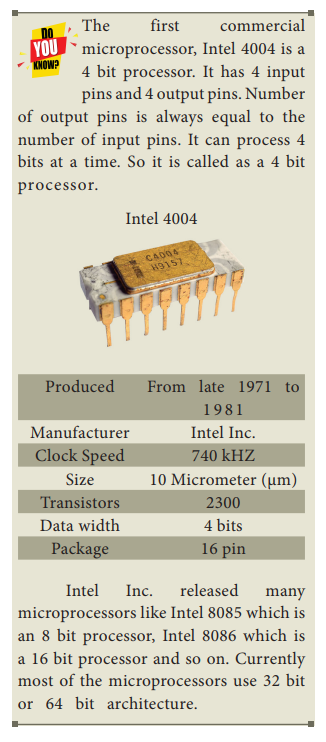Home | | Computer Technology 11th std | Characteristics of Microprocessors

# Characteristics of Microprocessors

A Microprocessor’s performance depends on the following characteristics:

Characteristics of Microprocessors

A Microprocessor’s performance depends on the following characteristics:

·     Clock speed

·     Instruction set

·     Word size

## a) Clock Speed

Every microprocessor has an internal clock that regulates the speed at which it executes instructions. The speed at which the microprocessor executes instructions is called the clock speed. Clock speed is measured in MHz (Mega Hertz) or in GHz (Giga Hertz).

## b) Instruction Set

A command which is given to a computer to perform an operation on  data is called an instruction. Basic set of machine level instructions that a microprocessor is designed to execute is called as an instruction set. This instruction set carries out the following types of operations:

•  Data transfer

•  Arithmetic operations

•  Logical operations

•  Control flow

•  Input/output

## c) Word Size

The number of bits that can be processed by a processor in a single instruction is called its word size. Word size determines the amount of RAM that can be accessed by a microprocessor at one time and the total number of pins on the microprocessor. Total number of input and output pins in turn determines the architecture of the microprocessor.

## Speed Measurement

Hertz – abbreviated as Hz is the standard unit of measurement used for measuring frequency.

Since frequency is measured in cycles per second, one hertz equals one cycle per second.

Hertz is commonly used to measure wave frequencies, such as sound waves, light waves, and radio waves. For example, the average human ear can detect sound waves between 20 and 20,000 Hz. Sound waves close to 20 Hz have a low pitch and are called "bass" frequencies. Sound waves above 5,000 Hz have a high pitch and are called "treble" frequencies.

While hertz can be used to measure wave frequencies, it is also used to measure the speed of computer processors. For example, each CPU is rated at a specific clock speed. This number indicates how many instruction cycles the processor can perform in every second. Since modern processors can perform millions or even billions of instructions per second, clock speeds are typically measured in megahertz or gigahertz.Study Material, Lecturing Notes, Assignment, Reference, Wiki description explanation, brief detail
11th Computer Science : Chapter 3 : Computer Organization : Characteristics of Microprocessors |